Home > CAAC > Chapter 5 > Lesson 5.1.3 > Problem5-30

5-30.
1. Review what you know about graphs by answering the following questions. 5-30 HW eTool (Desmos). Homework Help ✎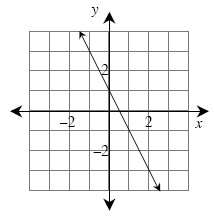1. Find the equation of the line graphed above.

2. What are its x- and y-intercepts?

3. On your own graph paper, graph the line.

4. On the same set of axes, graph a line parallel to the line graphed at right, but through the origin (0, 0). Find the equation of this new line.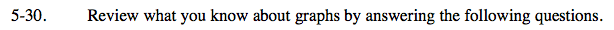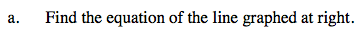Use the y-intercept and the growth rate to write the equation of the line at right.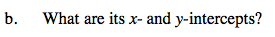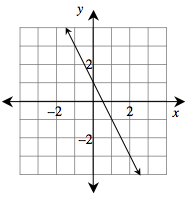Remember that the x-intercept is the point on the graph where y = 0 and that the y-intercept is the point where x = 0.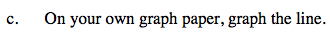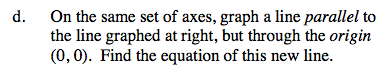Remember that parallel lines have the exact same growth rate.

Plug in the point given (0,0) into the basic equation y = mx + b and simplify to find the equation of the line.

m = the growth rate
b = the y-intercept

Use the eTool below to help you with this problem.
Click the link at right for the full version of the eTool: AC 5-30 HW eTool